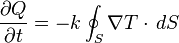Heat conduction facts for kids

Kids Encyclopedia Facts

Heat conduction (or thermal conduction) is the movement of heat from one solid to another one that has different temperature when they are touching each other. For example, we can warm our hands by touching hot-water bottles. When the cold hands touch the hot-water bottle, heat flows from the hotter object (hot-water bottle) to the colder one (hand).

Other ways to transfer heat are by thermal radiation and/or convection. Usually more than one of these processes happen at the same time.

Microscopic explanation

In the atomic theory solids, liquids and gases are made of tiny particles called "atoms". The temperature of the material measures how fast the atoms are moving and the heat measures the total amount of energy due to the vibration of the atoms.

You might imagine conduction to take place when one part of a material is heated. The atoms in this part vibrate faster and are more likely to hit their neighbours. When collisions take place, the energy is passed on to the neighbouring atoms allowing the energy to travel through the solid. (Rather like the way energy passes along a set of tumbling dominos).

The atomic picture also helps explain why conduction is more important in solids: in solids the atoms are close together and unable to move around. In liquids and gases the particles can move past each other, so the collisions are less common.

Law of heat conduction

The law of heat conduction, also known as Fourier's law, means that the rate, in time, of heat transfer through a material is proportional to the negative gradient in the temperature and to the area at right angles, to that gradient, through which the heat is flowing:$\frac{\partial Q}{\partial t} = -k \oint_S{\nabla T \cdot \,dS}$

where:

Q is the amount of heat transferred,
t is the time taken,
k is the material's thermal conductivity'
S is the area through which the heat is flowing,
T is the temperature.

Thermal conductivity usually varies with temperature, but the variation can be small, over a significant range of temperatures, for some common materials.

Other pagesHeat conduction Facts for Kids. Kiddle Encyclopedia.